## Selina Concise Mathematics Class 10 ICSE Solutions Chapter 1 Value Added Tax Ex 1A

These Solutions are part of Selina Concise Mathematics Class 10 ICSE Solutions. Here we have given Selina Concise Mathematics Class 10 ICSE Solutions Chapter 1 Value Added Tax Ex 1A.

Other Exercises

Question 1.
Rajat purchases a wrist-watch costing ₹ 540. The rate of Sales Tax is 8%. Find the total amount paid by Rajat for the watch.
Solution:
Cost of watch = ₹ 540
Rate of Sales Tax = 8%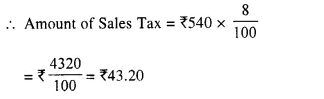Total Amount of Watch = ₹ 540 + ₹ 43.20 = ₹ 583.20

Question 2.
Ramesh paid ₹ 345.60 as Sales Tax on a purchase of ₹ 3,840. Find the rate of Sales Tax.
Solution:
On ₹ 3840, sales-tax is = ₹ 345.60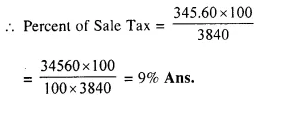Question 3.
The price of a washing machine, inclusive of sales tax is ₹ 13,530/-. If the Sales Tax is 10%, find its basic (cost) price. 
Solution:
Selling price of washing machine = ₹ 13,530.
Rate of Sales Tax = 10%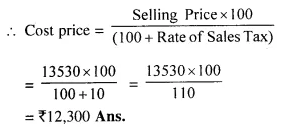Question 4.
Sarita purchases biscuits costing ₹ 158 on which the rate of Sales Tax is 6%. She also purchases some cosmetic goods costing ₹ 354 on which the rate of Sales Tax is 9%. Find the total amount to be paid by Sarita.
Solution:
Cost of biscuits = ₹ 158.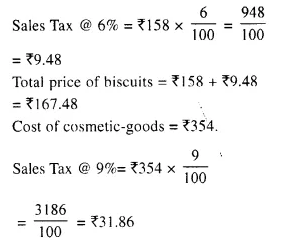Total cost of cosmetic goods = ₹ 354 + ₹ 31.86 = ₹ 385.86
Total amount paid by Sarita = ₹ 167. 48 + ₹ 385.86 = ₹ 553. 34

Question 5.
The marked price of two articles A and B together is ₹ 6,000. The sales tax on article A is 8% and that on article B is 10%. If on selling both the articles, the total sales tax collected is ₹ 552, find the marked price of each of the articles A and B.
Solution:
M.P of two articles A and B = ₹ 6000
Rate of S.T. on A = 8%
and rate of S.I. on B = 10%
Total sales tax on A and B = ₹ 552
Let MP of A be = ₹ x
and then of MP of B = ₹ (6000 – x)
according to the condition,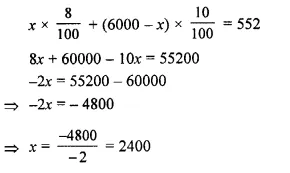M.P. of article A = ₹ 2400
and MP of article B = ₹ 6000 – ₹ 2400 = ₹ 3600

Question 6.
The price of a T.V. set inclusive of sales tax of 9% is ₹ 13,407. Find its marked price. If Sales Tax is increased to 13%, how much more does the customer has to pay for the T.V. ? 
Solution:
Sale price of T.V. set = ₹ 13,407
Rate of sales tax = 9%
Let marked price of T.V. = x
Then sale price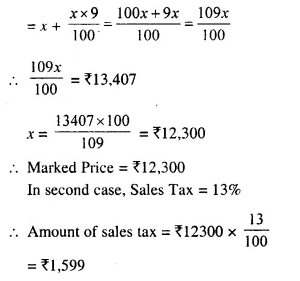Sale price = ₹ 12,300 + ₹ 1,599 = ₹ 13,899
Difference between the two sales price = ₹ 13,899 – ₹ 13,407 = ₹ 492

Question 7.
The price of an article is ₹ 8,250 which includes Sales Tax at 10%. Find how much more or less does a customer pay for the article, if the Sales Tax on the article:
(i) increases to 15%
(ii) decreases to 6%
(iii) increases by 2%
(iv) decreases by 3%
Solution:
Price of an article = ₹ 8,250
Rate of Sales Tax = 10%
Let the list price = x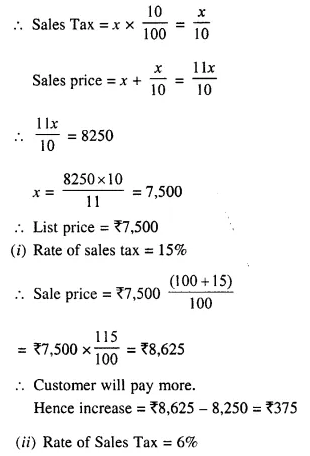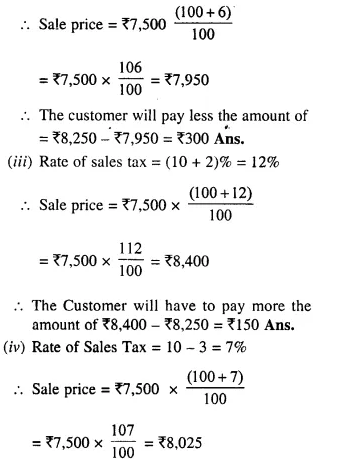The customer will have to pay less = ₹ 8,250 – ₹ 8,025 = ₹ 225

Question 8.
A bicycle is available for ₹ 1,664 including Sales Tax. If the list price of the bicycle is ₹ 1,600, find :
(i) the rate of Sales Tax
(ii) the price a customer will pay for the bicycle if the Sales Tax is increased by 6%.
Solution:
Sale price of bicycle = ₹ 1,664.
List price = ₹ 1,600
Amount of Sales Tax = ₹ 1,664 – ₹ 1,600 = ₹ 64.Sales price = ₹ 1,600 + ₹ 160 = ₹ 1,760

Question 9.
When the rate of sale-tax is decreased from 9% to 6% for a coloured T.V.; Mrs Geeta will save ₹ 780 in buying this T.V. Find the list price of the T.V.
Solution:
Rate of sales tax in the beginning = 9%
and Reduced rate = 6%
Diff. = 9 – 6 = 3%
Total saving = ₹ 780
List, price of TV = Total saving x $$\frac { 100 }{ 3 }$$
= $$\frac { 780 x 100 }{ 3 }$$ = ₹ 26000

Question 10.
A trader buys an unfinished article for ₹ 1,800 and spends ₹ 600 on its finishing, packing, transportation, etc. He marks the article at such a price that will give him 20% profit. How much will a customer pay for the article including 12% sales tax.
Solution:
Cost price of an unfinished article = ₹ 1800
Cost on finishing, packing etc or over head charges = ₹ 600
Total C.P. = ₹ 1800 + ₹ 600 = ₹ 2400
Gain = 20%Total sale price = ₹ 2880 + ₹ 345.60 = ₹ 3225.60

Question 11.
A shopkeeper buys an article for ₹ 800 and spends ₹ 100 on its transportation, etc. He marks the article at a certain price and then sells it Tor ₹ 1,287 including 10% sales tax. Find this profit as per cent.
Solution:
C.P. of an article = ₹ 800
Over head charges = ₹ 100
Total C.P. = ₹ 800 + ₹ 100 = ₹ 900
Sale price = ₹ 1287 including S.T.
Rate of S.T. = 10%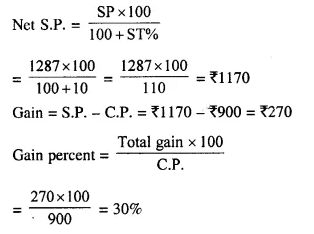Question 12.
A shopkeeper announces a discount of 15% on his goods. If the marked price of an article, in his shop, is ₹ 6000 ; how much a customer has to pay for it, if the rate of Sales Tax is 10% ?
Solution:
Marked price of an article = ₹ 6000
Rate of discount = 15%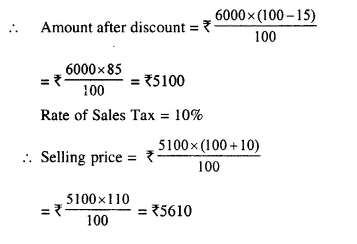Question 13.
The catalogue price of a music system is ₹ 24,000. The shopkeeper gives a discount of 8% on the list price. He gives a further off-season discount of 5% on the balance. But Sales Tax at 10% is charged on the remaining amount. Find:
(a) the Sales Tax a customer has to pay.
(b) the final price he has to pay for the music system. (2001)
Solution:
List price of colour music system = ₹ 24,000
First rate of discount = 8%(b) Final price of music system = ₹ 20,976 + ₹ 2,097.60 = ₹ 23,073.60

Hope given Selina Concise Mathematics Class 10 ICSE Solutions Chapter 1 Value Added Tax Ex 1A are helpful to complete your math homework.

If you have any doubts, please comment below. Learn Insta try to provide online math tutoring for you.